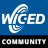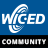cancel
Showing results for
Did you mean:

Wi-Fi ComboAnonymous
Not applicable

Second arg of:

What values are acceptable?

1 SolutionEmployee

Re: What is "sample_cycle" in wiced_adc_init()?

Hi,

Here is the logic to determine actual value used to set MCU peripheral:

/* Find the closest supported sampling time by the MCU */

for ( a = 0; ( a < sizeof( adc_sampling_cycle ) / sizeof(uint16_t) ) && adc_sampling_cycle < sample_cycle; a++ )

{

}

Seyhan

7 RepliesEmployee

Re: What is "sample_cycle" in wiced_adc_init()?

I don't know your exact answer, but (in SDK 3.1.1.) Line 106 of the file platform_adc.c (in the platform_adc_init() function), you can see the code step through an array of [supported] sample cycles.

{

};

Then, at line 111, it configures the ADC channel with the "found" value from the table.  Stated differently, if you pass in a garbage sample rate, the code self-protects itself to only sample at the supported rates.

So, I assume, the numbers in the table above are your choices.

I'm speculating on all of this.  What do you think?Employee

Re: What is "sample_cycle" in wiced_adc_init()?

An ADC is defined by its bandwidth (the range of frequencies it can measure) and its signal to noise ratio (how accurately it can measure a signal relative to the noise it introduces). The actual bandwidth of an ADC is characterized primarily by its sampling rate which is the sample cycle. Consider for instance 15 cycles is 4 MHz according to some specs . Based on the MCU you are using, you can refer to the data sheet can configure the number of cycles as Shawn has mentioned above.

Thnx

vik86Anonymous
Not applicable

Re: What is "sample_cycle" in wiced_adc_init()?

So the sample_cycle is the number of internal ADC clocks to take a single sample, correct?  When the comment

<snip>

If the MCU does not support the value provided, the closest supported value is used.

</snip>

Is closest based on delta from supported higher and lower values, or is there another means to determine the closest supported value?

Thanks, DenisEmployee

Re: What is "sample_cycle" in wiced_adc_init()?

Looking at the code, the comparison is a Less-Than operator.  Passing in a '52' would resolve down to a 28 per the table above.Anonymous
Not applicable

Re: What is "sample_cycle" in wiced_adc_init()?

Shawn,

you're right about the mechanism: the example from Broadcom asks for a "5", but internally a valid number which is close to the requested number is chosen. In this example, "5" is requested, "15" is programmed.

I will search the data sheet to know what those number mean in term of sample-per-second.Employee

Re: What is "sample_cycle" in wiced_adc_init()?

Hi,

Here is the logic to determine actual value used to set MCU peripheral:

/* Find the closest supported sampling time by the MCU */

for ( a = 0; ( a < sizeof( adc_sampling_cycle ) / sizeof(uint16_t) ) && adc_sampling_cycle < sample_cycle; a++ )

{

}

SeyhanAnonymous
Not applicable

Re: What is "sample_cycle" in wiced_adc_init()?